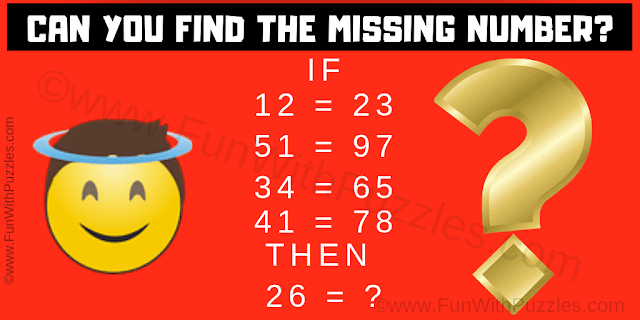It is Logical Reasoning Maths Brain Teaser which will Twist your Brain. In this Mind Twisting Logical Reasoning Brain Teaser, you are shown some logical number equations. These number equations follow a certain logical or mathematical pattern. Your challenge is to decode this relationship among the given numbers and then find the value of the missing number in the last equation which will replace the question mark.Can you find the missing number?
The answer to this "Logical Reasoning Maths Brain Teaser", can be viewed by clicking on the answer button.